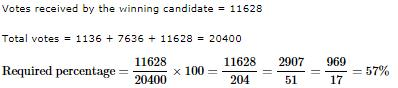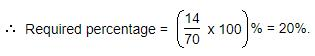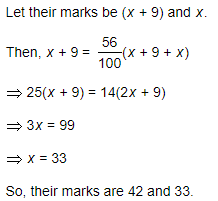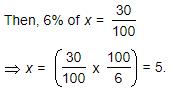Courses

# Test: Percentages- 2

## 10 Questions MCQ Test IBPS Clerk Prelims - Study Material, Mock Tests | Test: Percentages- 2

Description
This mock test of Test: Percentages- 2 for Quant helps you for every Quant entrance exam. This contains 10 Multiple Choice Questions for Quant Test: Percentages- 2 (mcq) to study with solutions a complete question bank. The solved questions answers in this Test: Percentages- 2 quiz give you a good mix of easy questions and tough questions. Quant students definitely take this Test: Percentages- 2 exercise for a better result in the exam. You can find other Test: Percentages- 2 extra questions, long questions & short questions for Quant on EduRev as well by searching above.
QUESTION: 1

### Three candidates contested an election and received 1136, 7636 and 11628 votes respectively. What percentage of the total votes did the winning candidate get?

Solution:QUESTION: 2

Solution:
QUESTION: 3

### What percentage of numbers from 1 to 70 have 1 or 9 in the unit's digit?

Solution:

Clearly, the numbers which have 1 or 9 in the unit's digit, have squares that end in the digit 1. Such numbers from 1 to 70 are 1, 9, 11, 19, 21, 29, 31, 39, 41, 49, 51, 59, 61, 69.

Number of such number =14QUESTION: 4

Two tailors X and Y are paid a total of Rs. 550 per week by their employer. If X is paid 120 percent of the sum paid to Y, how much is Y paid per week?

Solution:

X+ y=Rs. 550
x = 120% of y
120% * y+ y =550
120/100*y+y = 550
6/5y+y =550
6y+5y/5 = 550
11/5y = 550
11y = 550*5
y = 250

QUESTION: 5

Two numbers A and B are such that the sum of 5% of A and 4% of B is two-third of the sum of 6% of A and 8% of B. Find the ratio of A : B.

Solution:

5100A+4100B=23[6A100+8B100]

5A+4B=2/3(6A+8B)

15A+12B=12A+16B

3A=4B

A/B=4/3⇒

A : B = 4 : 3

QUESTION: 6

If 20% of a = b, then b% of 20 is the same as:

Solution:

20% of a = b  => (20/100)a = b

b% of 20 =(b/100) x 20 = (20a/100) x (1/100) x (20) = 4a/100 = 4% of a.

QUESTION: 7

In an election between two candidates, one got 55% of the total valid votes, 20% of the votes were invalid. If the total number of votes was 7500, the number of valid votes that the other candidate got, was:

Solution:

Total number of votes = 7500

Given that 20% of Percentage votes were invalid

1st candidate got 55% of the total valid votes.

Hence the 2nd candidate should have got 45% of the total valid votes

=> Valid votes that 2nd candidate got = total valid votes x (45/100)

7500*(80/100)*(45/100) = 2700

QUESTION: 8

Practice Quiz or MCQ (Multiple Choice Questions) with solution are available for Practice, which would help you prepare for Percentages under Quantitative Aptitude. You can practice these practice quizzes as per your speed and improvise the topic. The same topic is covered under various competitive examinations like - CAT, GMAT, Bank PO, SSC and other competitive examinations.

Q. Two students appeared at an examination. One of them secured 9 marks more than the other and his marks was 56% of the sum of their marks. The marks obtained by them are:

Solution:QUESTION: 9

A fruit seller had some apples. He sells 40% apples and still has 420 apples. Originally, he had:

Solution:

x-x(40/100)=420
x-40x/100=420
or, 60x/100=420
x=42000/60
x= 700

QUESTION: 10

Gauri went to the stationers and bought things worth Rs. 25, out of which 30 paise went on sales tax on taxable purchases. If the tax rate was 6%, then what was the cost of the tax free items?

Solution:

Let the amout taxable purchases be Rs. x.∴ Cost of tax free items = Rs. [25-(5+0.30)] = Rs. 19.70